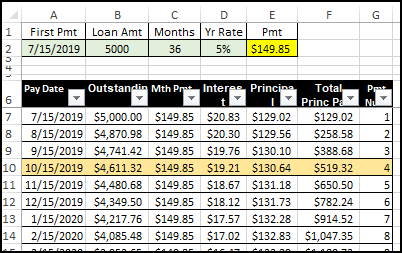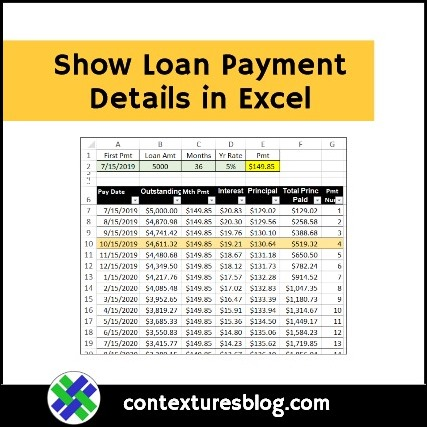## Show Loan Payments in Excel with PMT and IPMT

Written by Debra Dalgleish from Contextures Blog

With the PMT function, you can enter a few numbers, and calculate the monthly payments for a loan amount. But when is each payment due, and how much goes to interest and principal? This PMT and IPMT functions example shows how to calculate that too.

### PMT and IPMT Example

This example shows how to calculate monthly payments and interest amounts with the Excel PMT and IPMT functions.

Your loan company might use different calculations, so check with them for accurate numbers. This example is for entertainment purposes only!

### Loan Details

In this example, the loan details are entered at the top of the worksheet, and the PMT function will calculate the monthly payment amount.

There are 3 required numbers for the PMT function:• Rate: The annual rate is entered in cell D2 (named LoanRate)
• Periods: The number of months for the loan term is entered in cell C2 (named LoanMths)
• Value: The loan amount is entered in cell B2 (named LoanAmt)

The other green cell (A2, named LoanStart) is for the date of the first payment. It’s not required for the PMT function, but will be used in the payment table.

### Calculate Payment Amount

The PMT function is used in cell E2 (named LoanPmt), to calculate the monthly payment amount.

• =-PMT(LoanRate/12,LoanMths,LoanAmt)• There’s a minus sign at the start of the formula, to show the result as a positive number.
• The annual rate is divided by 12, to calculate the monthly rate

The PMT function calculates that if we borrow \$5000, over 36 months, at an annual rate of 5%, the monthly payment will be \$149.85

There are more PMT function examples on my Contextures website.

### Payment Details Table

Below the PMT calculation, there is a named Excel table – tblPay – which is filled with formulas. It will calculate the details for each payment.The table has 48 rows for monthly data. If you’re paying over a longer period, add more rows to the table. The formulas should fill down to the new rows automatically

### Payment Table Columns

There are 7 columns in the payment details table. Here are the column names and purpose:

• Pay Date: Payment due date
• Outstanding: Outstanding loan amount, before payment is made
• Mth Pmt: Payment amount
• Interest: Interest amount in the payment
• Principal: Principal amount in the payment
• Total Princ Paid: Running total of principal amount paid
• Pmt Num: Payment number

### Payment Number

In column G, this formula calculates the payment number, using the MAX function and SUM function:

=IF(MAX(G\$6:G6)< LoanMths, SUM(G6,1),””)

In this example, the loan term is 36 months, so the payment numbers stop at 36, in row 42.

• In row 43, the MAX in G\$6:G42 is 36.
• That is NOT less than the number in the LoanMths cell, so the result is an empty string### Other Formulas

All of the other formulas check the Pmt Num column ( G ), to see if it’s empty. If it is, the other formulas show an empty string too.

Here’s the start of all the other formulas:

=IF(G7=””,””,

### Pay Date Formula

In column A, this formula calculates each payment date, based on the start date entered in cell A1 (LoanStart):

=IF(G7=””,””,
DATE(YEAR(LoanStart),
MONTH(LoanStart)+G7-1,
DAY(LoanStart)))

The DATE function gets the year, month and day from the LoanStart cell.

For the month, it also adds the Payment number, and then subtracts 1.

So, if the first payment is December 1, 2019, the second payment is January 1, 2020.

DATE(2019, 12+2-1,1)Excel is smart enough to show that as a real date, instead of creating an error because we told it to show month 13 (12+2-1)

### Mth Pmt Formula

In column C, there’s a simple formula to show the monthly payment amount, based on the PMT function result at the top of the worksheet:

=IF(G7=””,””,LoanPmt)

### Interest Formula

In column D, the IPMT function calculates the interest amount in each payment:

=IF(G7=””,””,-IPMT(LoanRate/12, G7, LoanMths, LoanAmt))The IPMT function is similar to the PMT function, but uses the payment number as its second argument (G7)

Again, I put a minus sign in front of the function, to return a positive number.

### Principal  and Outstanding Formulas

After the interest is calculated, it’s easy to find the principal amount – just subtract the interest amount from the payment amount.

=IF(G7=””,””,C7-D7)

From the principal amounts, we can calculate the Outstanding amount. In each row, it’s the original loan amount, minus the total principal amounts in the rows above.

=IF(G7=””,””,LoanAmt-SUM(E\$6:E6))

And for the Total Princ Paid column, the formula is a total of all the principal amounts, including the current row.

=IF(G7=””,””,SUM(E\$6:E7))

This screen shot shows the formula in row 9, with the sum starting in the header cell (E\$6), and ending in E9### Conditional Formatting

There’s a conditional formatting rule on the table, so it highlights the latest payment, based on the current date.To create the conditional formatting rule, I followed these steps:

Select all the data cells in the table, starting from cell A7.

On the Excel Ribbon’s Home tab, click Conditional Formatting, then click New Rule

For Rule Type, select “Use a formula to determine which cells to format”

In the formula box, type this INDEX/MATCH formula, which refers to cell A7 (the active cell):

• =\$A7=INDEX(\$A\$7:\$A\$54, MATCH(TODAY(), \$A\$7:\$A\$54,1))

### How the Rule Works

In the Conditional Formatting rule:

• MATCH looks for the current date (TODAY function) in the payment date column
• If the current date is not found, MATCH returns the location of the latest date before the current date, because the 3rd argument is 1 (Less than)
• Then, the INDEX function returns the date from that location in the list of payment dates
• If the date in the current row matches that date, the table row is highlighted with light orange

### Get the Sample File

To see how the payment table works, you can download the completed sample file from my Contextures website.

On the PMT Function page, go to the download section, and get the Example #4 file.

The zipped file is in xlsx format, and does not contain any macros.

____________________

### Show Loan Payments in Excel____________________

Show Loan Payments in Excel with PMT and IPMT is a post from Contextures Blog and is not allowed to be copied to other sites